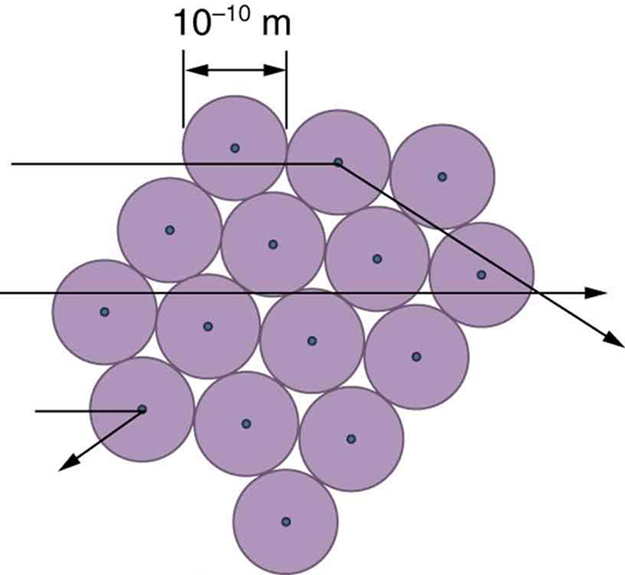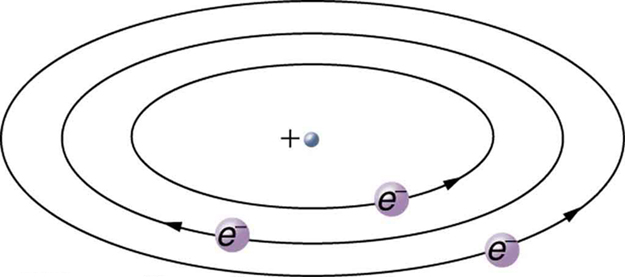# 30.2 Discovery of the parts of the atom: electrons and nuclei  (Page 5/7)

 Page 5 / 7

Although the results of the experiment were published by his colleagues in 1909, it took Rutherford two years to convince himself of their meaning. Like Thomson before him, Rutherford was reluctant to accept such radical results. Nature on a small scale is so unlike our classical world that even those at the forefront of discovery are sometimes surprised. Rutherford later wrote: “It was almost as incredible as if you fired a 15-inch shell at a piece of tissue paper and it came back and hit you. On consideration, I realized that this scattering backwards ... [meant] ... the greatest part of the mass of the atom was concentrated in a tiny nucleus.” In 1911, Rutherford published his analysis together with a proposed model of the atom. The size of the nucleus was determined to be about ${\text{10}}^{-\text{15}}\phantom{\rule{0.25em}{0ex}}\text{m}$ , or 100,000 times smaller than the atom. This implies a huge density, on the order of ${\text{10}}^{\text{15}}\phantom{\rule{0.25em}{0ex}}{\text{g/cm}}^{3}$ , vastly unlike any macroscopic matter. Also implied is the existence of previously unknown nuclear forces to counteract the huge repulsive Coulomb forces among the positive charges in the nucleus. Huge forces would also be consistent with the large energies emitted in nuclear radiation.

The small size of the nucleus also implies that the atom is mostly empty inside. In fact, in Rutherford’s experiment, most alphas went straight through the gold foil with very little scattering, since electrons have such small masses and since the atom was mostly empty with nothing for the alpha to hit. There were already hints of this at the time Rutherford performed his experiments, since energetic electrons had been observed to penetrate thin foils more easily than expected. [link] shows a schematic of the atoms in a thin foil with circles representing the size of the atoms (about ${\text{10}}^{-\text{10}}\phantom{\rule{0.25em}{0ex}}\text{m}$ ) and dots representing the nuclei. (The dots are not to scale—if they were, you would need a microscope to see them.) Most alpha particles miss the small nuclei and are only slightly scattered by electrons. Occasionally, (about once in 8000 times in Rutherford’s experiment), an alpha hits a nucleus head-on and is scattered straight backward.An expanded view of the atoms in the gold foil in Rutherford’s experiment. Circles represent the atoms (about 10 − 10 m size 12{"10" rSup { size 8{ - "10"} } " m"} {} in diameter), while the dots represent the nuclei (about 10 − 15 m size 12{"10" rSup { size 8{ - "15"} } " m"} {} in diameter). To be visible, the dots are much larger than scale. Most alpha particles crash through but are relatively unaffected because of their high energy and the electron’s small mass. Some, however, head straight toward a nucleus and are scattered straight back. A detailed analysis gives the size and mass of the nucleus.

Based on the size and mass of the nucleus revealed by his experiment, as well as the mass of electrons, Rutherford proposed the planetary model of the atom    . The planetary model of the atom pictures low-mass electrons orbiting a large-mass nucleus. The sizes of the electron orbits are large compared with the size of the nucleus, with mostly vacuum inside the atom. This picture is analogous to how low-mass planets in our solar system orbit the large-mass Sun at distances large compared with the size of the sun. In the atom, the attractive Coulomb force is analogous to gravitation in the planetary system. (See [link] .) Note that a model or mental picture is needed to explain experimental results, since the atom is too small to be directly observed with visible light.Rutherford’s planetary model of the atom incorporates the characteristics of the nucleus, electrons, and the size of the atom. This model was the first to recognize the structure of atoms, in which low-mass electrons orbit a very small, massive nucleus in orbits much larger than the nucleus. The atom is mostly empty and is analogous to our planetary system.

Rutherford’s planetary model of the atom was crucial to understanding the characteristics of atoms, and their interactions and energies, as we shall see in the next few sections. Also, it was an indication of how different nature is from the familiar classical world on the small, quantum mechanical scale. The discovery of a substructure to all matter in the form of atoms and molecules was now being taken a step further to reveal a substructure of atoms that was simpler than the 92 elements then known. We have continued to search for deeper substructures, such as those inside the nucleus, with some success. In later chapters, we will follow this quest in the discussion of quarks and other elementary particles, and we will look at the direction the search seems now to be heading.

## Phet explorations: rutherford scattering

How did Rutherford figure out the structure of the atom without being able to see it? Simulate the famous experiment in which he disproved the Plum Pudding model of the atom by observing alpha particles bouncing off atoms and determining that they must have a small core.

## Section summary

• Atoms are composed of negatively charged electrons, first proved to exist in cathode-ray-tube experiments, and a positively charged nucleus.
• All electrons are identical and have a charge-to-mass ratio of
$\frac{{q}_{e}}{{m}_{e}}=-\text{1.76}×{\text{10}}^{\text{11}}\phantom{\rule{0.25em}{0ex}}\text{C/kg.}$
• The positive charge in the nuclei is carried by particles called protons, which have a charge-to-mass ratio of
$\frac{{q}_{p}}{{m}_{p}}=9\text{.}\text{57}×{\text{10}}^{7}\phantom{\rule{0.25em}{0ex}}\text{C/kg}\text{.}$
• Mass of electron,
${m}_{e}=9\text{.}\text{11}×{\text{10}}^{-\text{31}}\phantom{\rule{0.25em}{0ex}}\text{kg}\text{.}$
• Mass of proton,
${m}_{p}=1\text{.}\text{67}×{\text{10}}^{-\text{27}}\phantom{\rule{0.25em}{0ex}}\text{kg.}$
• The planetary model of the atom pictures electrons orbiting the nucleus in the same way that planets orbit the sun.

## Conceptual questions

What two pieces of evidence allowed the first calculation of ${m}_{e}$ , the mass of the electron?

(a) The ratios ${q}_{e}/{m}_{e}$ and ${q}_{p}/{m}_{p}$ .

(b) The values of ${q}_{e}$ and ${E}_{B}$ .

(c) The ratio ${q}_{e}/{m}_{e}$ and ${q}_{e}$ .

How do the allowed orbits for electrons in atoms differ from the allowed orbits for planets around the sun? Explain how the correspondence principle applies here.

## Problem exercises

Rutherford found the size of the nucleus to be about ${\text{10}}^{-\text{15}}\phantom{\rule{0.25em}{0ex}}\text{m}$ . This implied a huge density. What would this density be for gold?

$\text{6}×{\text{10}}^{\text{20}}\phantom{\rule{0.25em}{0ex}}{\text{kg/m}}^{3}$

In Millikan’s oil-drop experiment, one looks at a small oil drop held motionless between two plates. Take the voltage between the plates to be 2033 V, and the plate separation to be 2.00 cm. The oil drop (of density $0\text{.}{\text{81 g/cm}}^{3}$ ) has a diameter of $4\text{.}0×{\text{10}}^{-6}\phantom{\rule{0.25em}{0ex}}\text{m}$ . Find the charge on the drop, in terms of electron units.

(a) An aspiring physicist wants to build a scale model of a hydrogen atom for her science fair project. If the atom is 1.00 m in diameter, how big should she try to make the nucleus?

(b) How easy will this be to do?

(a) $\text{10.0 μm}$

(b) It isn’t hard to make one of approximately this size. It would be harder to make it exactly $\text{10.0 μm}$ .

#### Questions & Answers

formula for impedance
ehat is central forces
what is distance?
What does mean ohms law imply
ohms law state that the electricity passing through a metallic conductor is directly proportional to the potential difference across its end
muyiwa
what is matter
Anything that occupies space
Kevin
Any thing that has weight and occupies space
Victoria
Anything which we can feel by any of our 5 sense organs
Suraj
Right
Roben
thanks
Suraj
what is a sulphate
Alo
Alo
the time rate of increase in velocity is called
acceleration
Emma
What is uniform velocity
Victoria
Greetings,users of that wonderful app.
how to solve pressure?
how do we calculate weight and eara eg an elefant that weight 2000kg has four fits or legs search of surface eara is 0.1m2(1metre square) incontact with the ground=10m2(g =10m2)
Cruz
P=F/A
Mira
can someone derive the formula a little bit deeper?
Bern
what is coplanar force?
forces acting and lying on d same plane
Promise
what is accuracy and precision
How does a current follow?
follow?
akif
which one dc or ac current.
akif
how does a current following?
Vineeta
?
akif
AC current
Vineeta
AC current follows due to changing electric field and magnetic field.
akif
you guys are just saying follow is flow not follow please
Abubakar
ok bro thanks
akif
flows
Abubakar
but i wanted to understand him/her in his own language
akif
but I think the statement is written in English not any other language
Abubakar
my mean that in which form he/she written this,will understand better in this form, i write.
akif
ok
Abubakar
ok thanks bro. my mistake
Vineeta
u are welcome
Abubakar
what is a semiconductor
substances having lower forbidden gap between valence band and conduction band
akif
what is a conductor?
Vineeta
replace lower by higher only
akif
convert 56°c to kelvin
Abubakar
How does a current follow?
Vineeta
A semiconductor is any material whose conduction lies between that of a conductor and an insulator.
AKOWUAH
what is Atom? what is molecules? what is ions?
atoms are the smallest unit of an element which is capable of behaving as a single unit
Promise
a molecule is d smallest unit of a substances capable of independent existence and can also retain the chemical proper ties of that substance
Promise
an ion is referred to as freely moving charged particles
Promise
What is a molecule
Is a unit of a compound that has two or more atoms either of the same or different atoms
Justice
A molecule is the smallest indivisible unit of a compound, Just like the atom is the smallest indivisible unit of an element.
Rachel
what is a molecule?
Vineeta
what is a vector
A quantity that has both a magnitude AND a direction. E.g velocity, acceleration, force are all vector quantities. Hope this helps :)
deage
what is the difference between velocity and relative velocity?
Mackson
Velocity is the rate of change of displacement with time. Relative velocity on the other hand is the velocity observed by an observer with respect to a reference point.
Chuks
what do u understand by Ultraviolet catastrophe?
Rufai
A certain freely falling object, released from rest, requires 1.5seconds to travel the last 30metres before it hits the ground. (a) Find the velocity of the object when it is 30metres above the ground.
Mackson
A vector is a quantity that has both magnitude and direction
Rufus
the velocity Is 20m/s-2
Rufus
what there factors affect the surface tension of a liquidByByBy Sam Luong